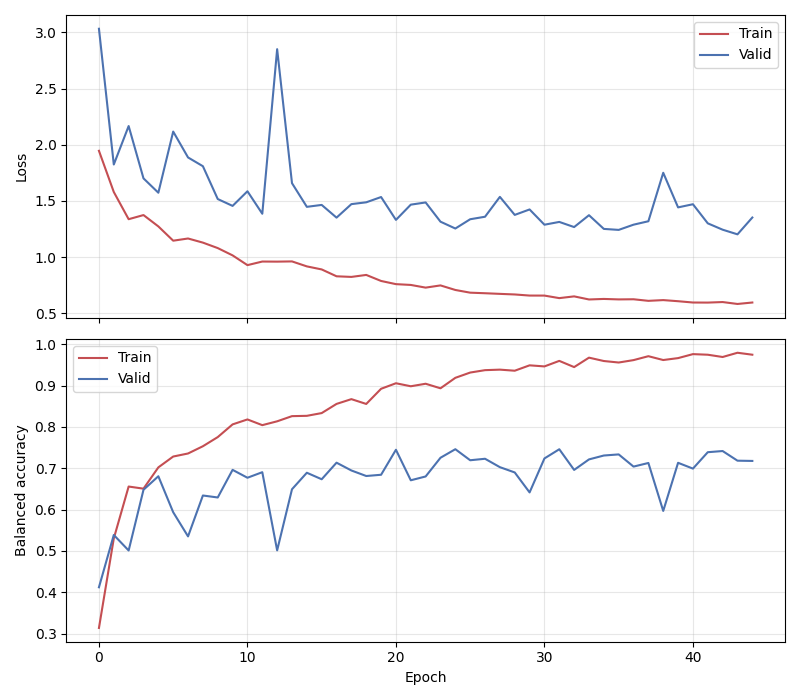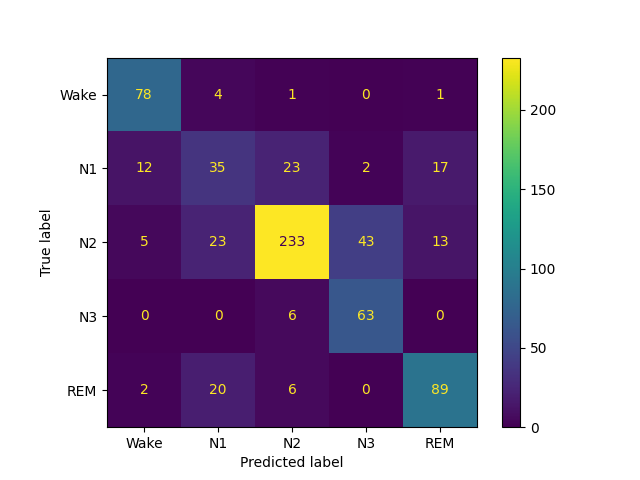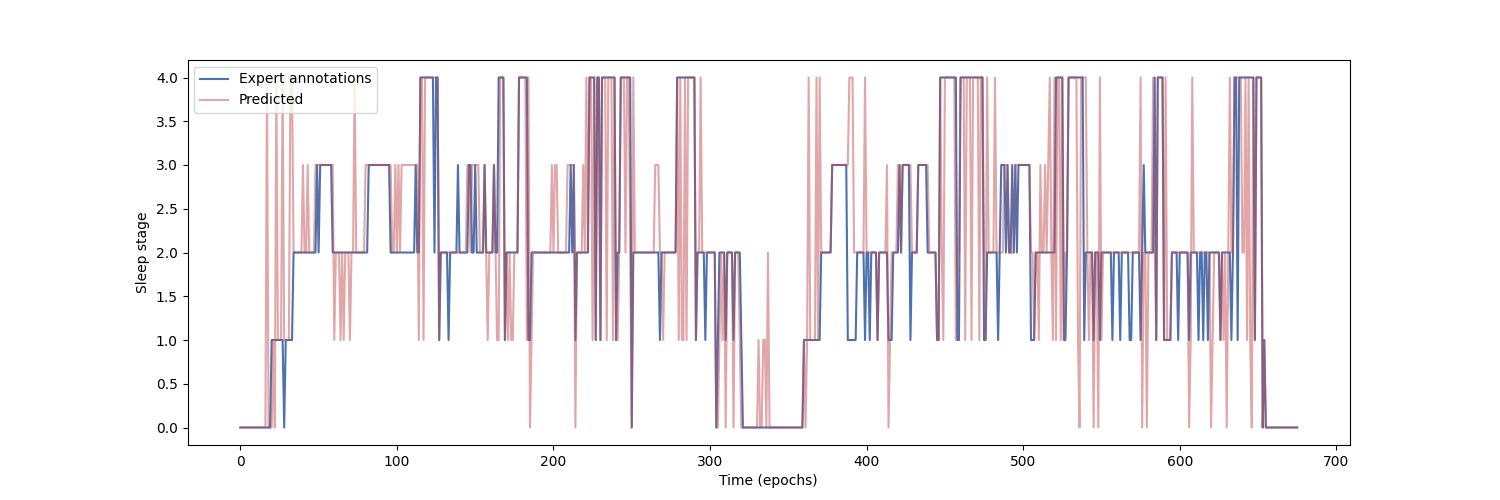# Sleep staging on the Sleep Physionet dataset using Eldele2021#

This tutorial shows how to train and test a sleep staging neural network with Braindecode. We use the attention-based model from  with the time distributed approach of  to learn on sequences of EEG windows using the openly accessible Sleep Physionet dataset  .

```# Authors: Divyesh Narayanan <divyesh.narayanan@gmail.com>
#
```

First, we load the data using the `braindecode.datasets.sleep_physionet.SleepPhysionet` class. We load two recordings from two different individuals: we will use the first one to train our network and the second one to evaluate performance (as in the MNE sleep staging example).

```from numbers import Integral
from braindecode.datasets import SleepPhysionet

subject_ids = [0, 1]
crop = (0, 30 * 400)  # we only keep 400 windows of 30s to speed example
dataset = SleepPhysionet(
subject_ids=subject_ids, recording_ids=, crop_wake_mins=30,
crop=crop)
```

### Preprocessing#

Next, we preprocess the raw data. We convert the data to microvolts and apply a lowpass filter.

```from braindecode.preprocessing import preprocess, Preprocessor
from numpy import multiply

high_cut_hz = 30
# Factor to convert from V to uV
factor = 1e6

preprocessors = [
Preprocessor(lambda data: multiply(data, factor), apply_on_array=True),  # Convert from V to uV
Preprocessor('filter', l_freq=None, h_freq=high_cut_hz)
]

# Transform the data
preprocess(dataset, preprocessors)
```
```/home/runner/work/braindecode/braindecode/braindecode/preprocessing/preprocess.py:55: UserWarning: Preprocessing choices with lambda functions cannot be saved.
warn('Preprocessing choices with lambda functions cannot be saved.')

<braindecode.datasets.sleep_physionet.SleepPhysionet object at 0x7f4526d57220>
```

### Extract windows#

We extract 30-s windows to be used in the classification task. The Eldele2021 model takes a single channel as input. Here, the Fpz-Cz channel is used as it was found to give better performance than using the Pz-Oz channel

```from braindecode.preprocessing import create_windows_from_events

mapping = {  # We merge stages 3 and 4 following AASM standards.
'Sleep stage W': 0,
'Sleep stage 1': 1,
'Sleep stage 2': 2,
'Sleep stage 3': 3,
'Sleep stage 4': 3,
'Sleep stage R': 4
}

window_size_s = 30
sfreq = 100
window_size_samples = window_size_s * sfreq

windows_dataset = create_windows_from_events(
dataset,
trial_start_offset_samples=0,
trial_stop_offset_samples=0,
window_size_samples=window_size_samples,
window_stride_samples=window_size_samples,
picks="Fpz-Cz",  # the other option is Pz-Oz,
mapping=mapping
)
```
```/home/runner/work/braindecode/braindecode/braindecode/preprocessing/windowers.py:130: UserWarning: Using reject or picks or flat or dropping bad windows means mne Epochs are created, which will be substantially slower and may be deprecated in the future.
warnings.warn('Using reject or picks or flat or dropping bad windows means '
```

### Window preprocessing#

We also preprocess the windows by applying channel-wise z-score normalization in each window.

```from sklearn.preprocessing import scale as standard_scale

preprocess(windows_dataset, [Preprocessor(standard_scale, channel_wise=True)])
```
```<braindecode.datasets.base.BaseConcatDataset object at 0x7f4526d14220>
```

### Split dataset into train and valid#

We split the dataset into training and validation set taking every other subject as train or valid.

```split_ids = dict(train=subject_ids[::2], valid=subject_ids[1::2])
splits = windows_dataset.split(split_ids)
train_set, valid_set = splits["train"], splits["valid"]
```

## Create sequence samplers#

Following the time distributed approach of , we need to provide our neural network with sequences of windows, such that the embeddings of multiple consecutive windows can be concatenated and provided to a final classifier. We can achieve this by defining Sampler objects that return sequences of window indices. To simplify the example, we train the whole model end-to-end on sequences, rather than using the two-step approach of  (i.e. training the feature extractor on single windows, then freezing its weights and training the classifier).

```from braindecode.samplers import SequenceSampler

n_windows = 3  # Sequences of 3 consecutive windows
n_windows_stride = 3  # Maximally overlapping sequences

train_sampler = SequenceSampler(
)

# Print number of examples per class
print('Training examples: ', len(train_sampler))
print('Validation examples: ', len(valid_sampler))
```
```Training examples:  133
Validation examples:  133
```

We also implement a transform to extract the label of the center window of a sequence to use it as target.

```import numpy as np

# Use label of center window in the sequence
def get_center_label(x):
if isinstance(x, Integral):
return x
return x[np.ceil(len(x) / 2).astype(int)] if len(x) > 1 else x

train_set.target_transform = get_center_label
valid_set.target_transform = get_center_label
```

Finally, since some sleep stages appear a lot more often than others (e.g. most of the night is spent in the N2 stage), the classes are imbalanced. To avoid overfitting on the more frequent classes, we compute weights that we will provide to the loss function when training.

```from sklearn.utils import compute_class_weight

y_train = [train_set[idx] for idx in train_sampler]
class_weights = compute_class_weight('balanced', classes=np.unique(y_train), y=y_train)
```

## Create model#

We can now create the deep learning model. In this tutorial, we use the sleep staging architecture introduced in , which is an attention-based neural network. We use the time distributed version of the model, where the feature vectors of a sequence of windows are concatenated and passed to a linear layer for classification.

```import torch
from torch import nn
from braindecode.util import set_random_seeds
from braindecode.models import SleepStagerEldele2021, TimeDistributed

cuda = torch.cuda.is_available()  # check if GPU is available
device = 'cuda' if torch.cuda.is_available() else 'cpu'
if cuda:
torch.backends.cudnn.benchmark = True
# Set random seed to be able to reproduce results
set_random_seeds(seed=31, cuda=cuda)

n_classes = 5
# Extract number of channels and time steps from dataset
n_channels, input_size_samples = train_set.shape

feat_extractor = SleepStagerEldele2021(
sfreq,
n_outputs=n_classes,
n_times=input_size_samples,
return_feats=True,
)

model = nn.Sequential(
TimeDistributed(feat_extractor),  # apply model on each 30-s window
nn.Sequential(  # apply linear layer on concatenated feature vectors
nn.Flatten(start_dim=1),
nn.Dropout(0.5),
nn.Linear(feat_extractor.len_last_layer * n_windows, n_classes)
)
)

# Send model to GPU
if cuda:
model.cuda()
```

## Training#

We can now train our network. `braindecode.EEGClassifier` is a braindecode object that is responsible for managing the training of neural networks. It inherits from `skorch.NeuralNetClassifier`, so the training logic is the same as in Skorch.

```from skorch.helper import predefined_split
from skorch.callbacks import EpochScoring
from braindecode import EEGClassifier

lr = 1e-3
batch_size = 32
n_epochs = 3  # we use few epochs for speed and but more than one for plotting

train_bal_acc = EpochScoring(
scoring='balanced_accuracy', on_train=True, name='train_bal_acc',
lower_is_better=False)
valid_bal_acc = EpochScoring(
scoring='balanced_accuracy', on_train=False, name='valid_bal_acc',
lower_is_better=False)
callbacks = [
('train_bal_acc', train_bal_acc),
('valid_bal_acc', valid_bal_acc)
]

clf = EEGClassifier(
model,
criterion=torch.nn.CrossEntropyLoss,
criterion__weight=torch.Tensor(class_weights).to(device),
iterator_train__shuffle=False,
iterator_train__sampler=train_sampler,
iterator_valid__sampler=valid_sampler,
train_split=predefined_split(valid_set),  # using valid_set for validation
optimizer__lr=lr,
batch_size=batch_size,
callbacks=callbacks,
device=device,
classes=np.unique(y_train)
)
# Model training for a specified number of epochs. `y` is None as it is already
# supplied in the dataset.
clf.fit(train_set, y=None, epochs=n_epochs)
```
```  epoch    train_bal_acc    train_loss    valid_acc    valid_bal_acc    valid_loss     dur
-------  ---------------  ------------  -----------  ---------------  ------------  ------
1           0.1471        3.1815       0.3459           0.1795        3.4748  2.2577
2           0.2712        1.8805       0.1955           0.2186        2.1537  1.9869
3           0.4550        1.4941       0.2256           0.2200        4.2159  2.0100

<class 'braindecode.classifier.EEGClassifier'>[initialized](
module_=Sequential(
(0): TimeDistributed(
(module): SleepStagerEldele2021(
(feature_extractor): Sequential(
(0): _MRCNN(
(GELU): GELU(approximate='none')
(features1): Sequential(
(0): Conv1d(1, 64, kernel_size=(50,), stride=(6,), padding=(24,), bias=False)
(1): BatchNorm1d(64, eps=1e-05, momentum=0.1, affine=True, track_running_stats=True)
(2): GELU(approximate='none')
(3): MaxPool1d(kernel_size=8, stride=2, padding=4, dilation=1, ceil_mode=False)
(4): Dropout(p=0.5, inplace=False)
(5): Conv1d(64, 128, kernel_size=(8,), stride=(1,), padding=(4,), bias=False)
(6): BatchNorm1d(128, eps=1e-05, momentum=0.1, affine=True, track_running_stats=True)
(7): GELU(approximate='none')
(8): Conv1d(128, 128, kernel_size=(8,), stride=(1,), padding=(4,), bias=False)
(9): BatchNorm1d(128, eps=1e-05, momentum=0.1, affine=True, track_running_stats=True)
(10): GELU(approximate='none')
(11): MaxPool1d(kernel_size=4, stride=4, padding=2, dilation=1, ceil_mode=False)
)
(features2): Sequential(
(0): Conv1d(1, 64, kernel_size=(400,), stride=(50,), padding=(200,), bias=False)
(1): BatchNorm1d(64, eps=1e-05, momentum=0.1, affine=True, track_running_stats=True)
(2): GELU(approximate='none')
(3): MaxPool1d(kernel_size=4, stride=2, padding=2, dilation=1, ceil_mode=False)
(4): Dropout(p=0.5, inplace=False)
(5): Conv1d(64, 128, kernel_size=(7,), stride=(1,), padding=(3,), bias=False)
(6): BatchNorm1d(128, eps=1e-05, momentum=0.1, affine=True, track_running_stats=True)
(7): GELU(approximate='none')
(8): Conv1d(128, 128, kernel_size=(7,), stride=(1,), padding=(3,), bias=False)
(9): BatchNorm1d(128, eps=1e-05, momentum=0.1, affine=True, track_running_stats=True)
(10): GELU(approximate='none')
(11): MaxPool1d(kernel_size=2, stride=2, padding=1, dilation=1, ceil_mode=False)
)
(dropout): Dropout(p=0.5, inplace=False)
(AFR): Sequential(
(0): _SEBasicBlock(
(conv1): Conv1d(128, 30, kernel_size=(1,), stride=(1,))
(bn1): BatchNorm1d(30, eps=1e-05, momentum=0.1, affine=True, track_running_stats=True)
(relu): ReLU(inplace=True)
(conv2): Conv1d(30, 30, kernel_size=(1,), stride=(1,))
(bn2): BatchNorm1d(30, eps=1e-05, momentum=0.1, affine=True, track_running_stats=True)
(se): _SELayer(
(fc): Sequential(
(0): Linear(in_features=30, out_features=1, bias=False)
(1): ReLU(inplace=True)
(2): Linear(in_features=1, out_features=30, bias=False)
(3): Sigmoid()
)
)
(downsample): Sequential(
(0): Conv1d(128, 30, kernel_size=(1,), stride=(1,), bias=False)
(1): BatchNorm1d(30, eps=1e-05, momentum=0.1, affine=True, track_running_stats=True)
)
(features): Sequential(
(0): Conv1d(128, 30, kernel_size=(1,), stride=(1,))
(1): BatchNorm1d(30, eps=1e-05, momentum=0.1, affine=True, track_running_stats=True)
(2): ReLU(inplace=True)
(3): Conv1d(30, 30, kernel_size=(1,), stride=(1,))
(4): BatchNorm1d(30, eps=1e-05, momentum=0.1, affine=True, track_running_stats=True)
(5): _SELayer(
(fc): Sequential(
(0): Linear(in_features=30, out_features=1, bias=False)
(1): ReLU(inplace=True)
(2): Linear(in_features=1, out_features=30, bias=False)
(3): Sigmoid()
)
)
)
)
)
)
(1): _TCE(
(layers): ModuleList(
(0-1): 2 x _EncoderLayer(
(convs): ModuleList(
(0-2): 3 x _CausalConv1d(30, 30, kernel_size=(7,), stride=(1,), padding=(6,))
)
(linear): Linear(in_features=80, out_features=80, bias=True)
(dropout): Dropout(p=0.1, inplace=False)
)
(feed_forward): _PositionwiseFeedForward(
(w_1): Linear(in_features=80, out_features=120, bias=True)
(w_2): Linear(in_features=120, out_features=80, bias=True)
(dropout): Dropout(p=0.1, inplace=False)
)
(sublayer_output): ModuleList(
(0-1): 2 x _SublayerOutput(
(norm): LayerNorm((80,), eps=1e-06, elementwise_affine=True)
(dropout): Dropout(p=0.1, inplace=False)
)
)
(conv): _CausalConv1d(30, 30, kernel_size=(7,), stride=(1,), padding=(6,))
)
)
(norm): LayerNorm((80,), eps=1e-06, elementwise_affine=True)
)
)
)
)
(1): Sequential(
(0): Flatten(start_dim=1, end_dim=-1)
(1): Dropout(p=0.5, inplace=False)
(2): Linear(in_features=7200, out_features=5, bias=True)
)
),
)
```

## Plot results#

We use the history stored by Skorch during training to plot the performance of the model throughout training. Specifically, we plot the loss and the balanced balanced accuracy for the training and validation sets.

```import matplotlib.pyplot as plt
import pandas as pd

# Extract loss and balanced accuracy values for plotting from history object
df = pd.DataFrame(clf.history.to_list())
df.index.name = "Epoch"
fig, (ax1, ax2) = plt.subplots(2, 1, figsize=(8, 7), sharex=True)
df[['train_loss', 'valid_loss']].plot(color=['r', 'b'], ax=ax1)
df[['train_bal_acc', 'valid_bal_acc']].plot(color=['r', 'b'], ax=ax2)
ax1.set_ylabel('Loss')
ax2.set_ylabel('Balanced accuracy')
ax1.legend(['Train', 'Valid'])
ax2.legend(['Train', 'Valid'])
fig.tight_layout()
plt.show()
```Finally, we also display the confusion matrix and classification report:

```from sklearn.metrics import confusion_matrix, classification_report
from braindecode.visualization import plot_confusion_matrix

y_true = [valid_set[[i]] for i in range(len(valid_sampler))]
y_pred = clf.predict(valid_set)

confusion_mat = confusion_matrix(y_true, y_pred)

plot_confusion_matrix(confusion_mat=confusion_mat)
#                      class_names=['Wake', 'N1', 'N2', 'N3', 'N4', 'REM'])

print(classification_report(y_true, y_pred))
``````/home/runner/.local/lib/python3.10/site-packages/sklearn/metrics/_classification.py:1471: UndefinedMetricWarning: Precision and F-score are ill-defined and being set to 0.0 in labels with no predicted samples. Use `zero_division` parameter to control this behavior.
_warn_prf(average, modifier, msg_start, len(result))
/home/runner/.local/lib/python3.10/site-packages/sklearn/metrics/_classification.py:1471: UndefinedMetricWarning: Recall and F-score are ill-defined and being set to 0.0 in labels with no true samples. Use `zero_division` parameter to control this behavior.
_warn_prf(average, modifier, msg_start, len(result))
/home/runner/.local/lib/python3.10/site-packages/sklearn/metrics/_classification.py:1471: UndefinedMetricWarning: Precision and F-score are ill-defined and being set to 0.0 in labels with no predicted samples. Use `zero_division` parameter to control this behavior.
_warn_prf(average, modifier, msg_start, len(result))
/home/runner/.local/lib/python3.10/site-packages/sklearn/metrics/_classification.py:1471: UndefinedMetricWarning: Recall and F-score are ill-defined and being set to 0.0 in labels with no true samples. Use `zero_division` parameter to control this behavior.
_warn_prf(average, modifier, msg_start, len(result))
/home/runner/.local/lib/python3.10/site-packages/sklearn/metrics/_classification.py:1471: UndefinedMetricWarning: Precision and F-score are ill-defined and being set to 0.0 in labels with no predicted samples. Use `zero_division` parameter to control this behavior.
_warn_prf(average, modifier, msg_start, len(result))
/home/runner/.local/lib/python3.10/site-packages/sklearn/metrics/_classification.py:1471: UndefinedMetricWarning: Recall and F-score are ill-defined and being set to 0.0 in labels with no true samples. Use `zero_division` parameter to control this behavior.
_warn_prf(average, modifier, msg_start, len(result))
precision    recall  f1-score   support

0       1.00      0.02      0.03        60
1       0.00      0.00      0.00         6
2       0.00      0.00      0.00        48
3       0.15      1.00      0.26        19
4       0.00      0.00      0.00         0

accuracy                           0.15       133
macro avg       0.23      0.20      0.06       133
weighted avg       0.47      0.15      0.05       133
```

Finally, we can also visualize the hypnogram of the recording we used for validation, with the predicted sleep stages overlaid on top of the true sleep stages. We can see that the model cannot correctly identify the different sleep stages with this amount of training.

```import matplotlib.pyplot as plt

fig, ax = plt.subplots(figsize=(15, 5))
ax.plot(y_true, color='b', label='Expert annotations')
ax.plot(y_pred.flatten(), color='r', label='Predict annotations', alpha=0.5)
ax.set_xlabel('Time (epochs)')
ax.set_ylabel('Sleep stage')
``````Text(150.22222222222223, 0.5, 'Sleep stage')
```

The model was able to learn despite the low amount of data that was available (only two recordings in this example) and reached a balanced accuracy of about 43% in a 5-class classification task (chance-level = 20%) on held-out data over 10 epochs.

Note

To further improve performance, the number of epochs should be increased. It has been reduced here for faster run-time in document generation. In testing, 10 epochs provided reasonable performance with around 89% balanced accuracy on training data and around 43% on held out validation data. Increasing the number of training recordings and optimizing the hyperparameters will also help increase performance